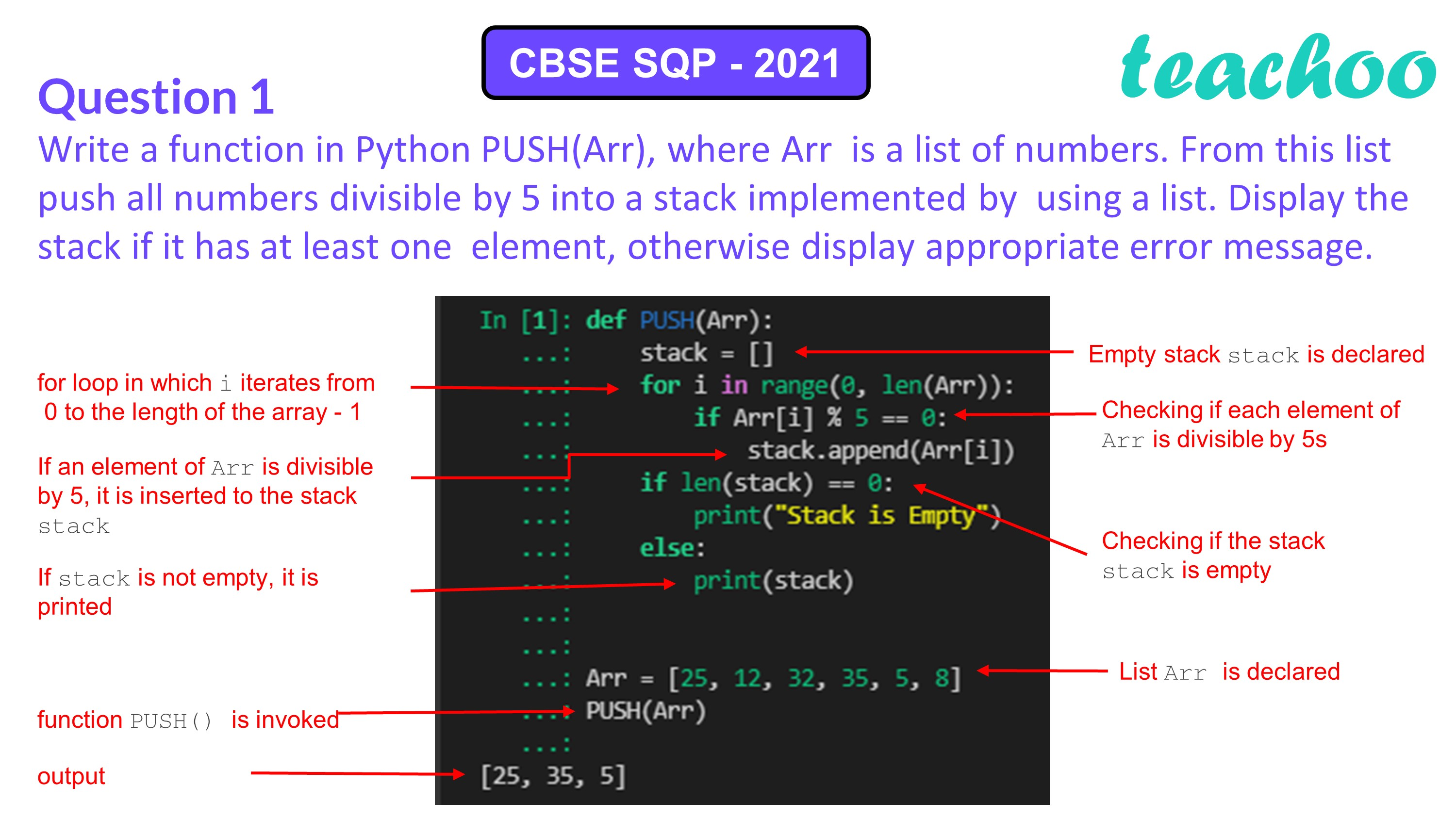Past Year - 3 Mark Questions

Computer Science - Class 12
Chapter 3 - Stacks

## Write a function in Python PUSH(Arr), where Arr is a list of numbers. From this list push all numbers divisible by 5 into a stack implemented by using a list. Display the stack if it has at least one element, otherwise display appropriate error message.

``` def PUSH(Arr): ```

``` stack=[] ```

``` for i in range(0,len(Arr)): ```

``` if Arr[i]%5==0: ```

``` stack.append(Arr[i]) ```

``` if len(stack)==0: ```

``` print("Stack is Empty") ```

``` else: ```

``` print(stack) ```Learn in your speed, with individual attention - Teachoo Maths 1-on-1 Class

### Transcript

Write a function in Python PUSH(Arr), where Arr is a list of numbers. From this list push all numbers divisible by 5 into a stack implemented by using a list. Display the stack if it has at least one element, otherwise display appropriate error message. Answer: def PUSH(Arr): stack=[] for i in range(0,len(Arr)): if Arr[i]%5==0: stack.append(Arr[i]) if len(stack)==0: print("Stack is Empty") else: print(stack)